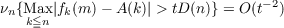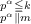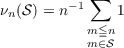#### Vol. 97, No. 2, 1981

 Recent Issues Vol. 325: 1 Vol. 324: 1  2 Vol. 323: 1  2 Vol. 322: 1  2 Vol. 321: 1  2 Vol. 320: 1  2 Vol. 319: 1  2 Vol. 318: 1  2Online Archive Volume: Issue:The Journal Subscriptions Editorial Board Officers Contacts Submission Guidelines Submission Form Policies for Authors ISSN: 1945-5844 (e-only) ISSN: 0030-8730 (print) Special Issues Author Index To Appear Other MSP Journals
An analogue of Kolmogorov’s inequality for a class of additive arithmetic functions

### Joseph Eugene Collison

Vol. 97 (1981), No. 2, 319–325
##### Abstract

Let f be a complex valued additive number theoretic function (i.e., f(mn) = f(m) + f(n) if m and n are relatively prime). This paper shows that D2(pα)pα = O(D2(n)) or |f(pα)|pα = O(D(n)) (where the summations are over those pα n, pα being a prime raised to a power) is sufficient to guarantee that the following analogue of Kolmogorov’s inequality holds:where, if pαm denotes the fact that pα divides m but pα+1 does not (i.e., pα exactly divides m), then

 A(n) = ∑ pα≦nf(pα)p−α, D2(n) = ∑ pα≦n|f(pα)|2p−α, fk(m) = ∑f(pα),
andfor any set 𝒮.

##### Mathematical Subject Classification
Primary: 10H25, 10H25
Secondary: 10K20
##### Milestones